Select Page

# CBSE Relations and Functions Maths 12 Science Answers for MCQ in English

CBSE Relations and Functions Maths 12 Science Answers for MCQ in English to enable students to get Answers in a narrative video format for the specific question.

Expert Teacher provides CBSE Relations and Functions Maths 12 Science Answers for MCQ through Video Answers in English language. This video solution will be useful for students to understand how to write an answer in exam in order to score more marks. This teacher uses a narrative style for a question from Relations and Functions not only to explain the proper method of answering question, but deriving right answer too.

Please find the question below and view the Answer in a narrative video format.

Question:

## Similar Questions from CBSE, 12th Science, Maths, Relations and Functions

Question 1 : A function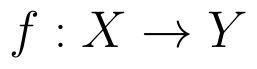is bijective if and only if, (View Answer Video)

Question 2 : Functions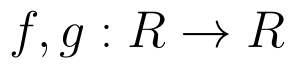are defined respectively, by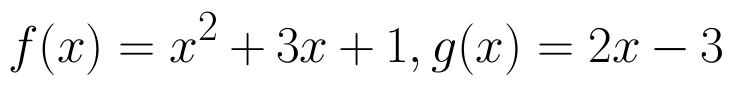, find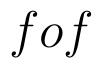(View Answer Video)

Question 3 : Let * be the binary operation on N given by a * b = LCM of a and b. Find 5 * 7. (View Answer Video)

Question 4 : Number of binary operations on the set { a, b} are: (View Answer Video)

Question 5 : Let A = {1, 2, 3}. Then, number of relations containing (1, 2) and (1, 3) which are reflexive and symmetric but not transitive is, (View Answer Video)

### Application of Integrals

Question 1 : Using integration, find the area of the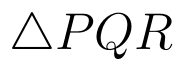co-ordinates whose vertices are P(2,0), Q(4, 5) and R(6,3). (View Answer Video)

Question 2 : A farmer has a field of shape bounded by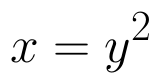and x = 3, he wants to divide this into his two sons equally by a straight line x = c. Can you find c? What value, you find in the person ? (View Answer Video)

Question 3 : Using the method of integration, find the area of the region bounded by the lines 3x - 2y + 1 = 0, 2x + 3y -21 = 0 and x - 5y + 9 = 0. (View Answer Video)

Question 4 : Using the method of integration find the area of the triangle ABC, coordinates of whose vertices are A(2, 0), B(4, 5) and C(6, 3). (View Answer Video)

Question 5 : Find the area of the circle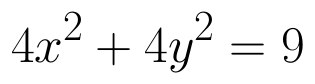which is interior to the parabola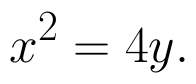(View Answer Video)

### Application of Derivatives

Question 1 : A tank with a rectangular base and rectangular sides, open at the top is to be constructed so that its depth is 2m and volume is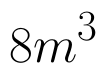If the building of tank costs Rs.70 per sq meter for the base and Rs.45 per sq meter for sides. What is the cost of least expensive? (View Answer Video)

Question 2 : What is the maximum value of the function sin x+ cos x? (View Answer Video)

Question 3 : Find the approximate change in the surface area of a cube of side x meters caused by decreasing the side by 1%. (View Answer Video)

Question 4 : Find the approximate value of f(5.001) where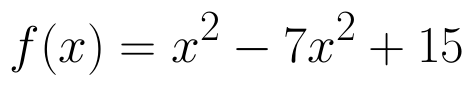. (View Answer Video)

Question 5 : The maximum value of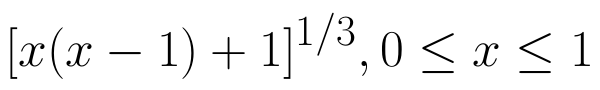is (View Answer Video)

### Vector Algebra

Question 1 : Find the unit vector in the direction of vector PQ, where P and Q are the points (1, 2, 3) and (4, 5, 6) respectively. (View Answer Video)

Question 2 : Find a unit vector in the direction of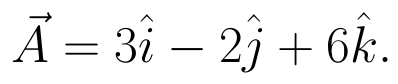(View Answer Video)

Question 3 :  Find the sum of the vectors :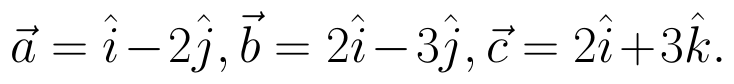(View Answer Video)

Question 4 : For given vectors,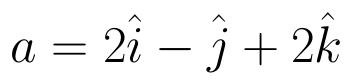and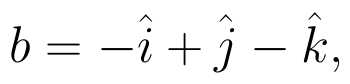find the unit vector in the direction of the vector a + b. (View Answer Video)

Question 5 : Find the magnitude of two vectors a and b having the same magnitude and such that the angle between them is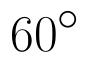and their scalar product is 1/2. (View Answer Video)